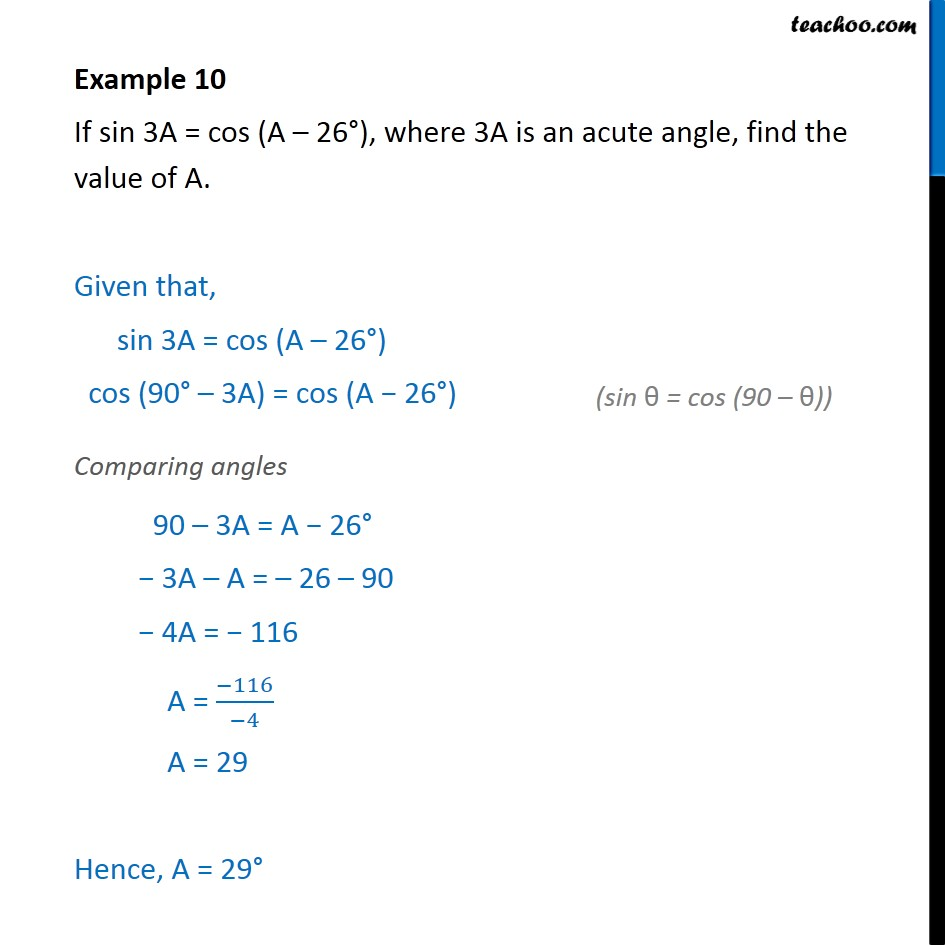1. Chapter 8 Class 10 Introduction to Trignometry
2. Serial order wise
3. Examples

Transcript

Example 10 If sin 3A = cos (A – 26°), where 3A is an acute angle, find the value of A. Given that, sin 3A = cos (A – 26°) cos (90° – 3A) = cos (A − 26°) Comparing angles 90 – 3A = A − 26° − 3A – A = – 26 – 90 − 4A = − 116 A = (−116)/(−4) A = 29 Hence, A = 29°

Examples